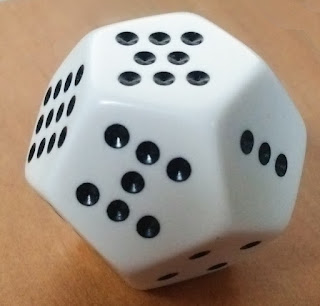## Saturday 22 May 2021You can mark two twelve sided dice to make them able to cast lines with the yarrow stalks probabilities.

One of the dice has:

• Three faces with 3 dots
• All the other faces with 4 dots.

The other dice has:

• Three faces with 3 dots
• Three faces with 5 dots
• All the other faces with 4 dots.

To cast a line you just cast the two dice together and sum the dots.

You'll get 6,7,8 or 9 with yarrow stalks probabilites:

#### Probabilities

The table on the right shows all the possible combinations for the two dice. Counting the number of 6,7,8 and 9 it's easy to see that:

Prob(6) = 9/144 = 1/16
Prob(8) = 63/144 = 7/16
Prob(7) = 45/144 = 5/16
Prob(9) = 27/144 = 3/16
Prob(yin) = Prob(yang) = 1/2

#### Variations

To get lines with 3 coins probability, mark the first dice as follows:

• Six faces with 3 dots
• Six faces with 4 dots